Sale ends in
Teacher: Kristi
Customers Who Have Viewed This Course: 1454
\$225.00
\$112.00

#### 0 Introduction 02:16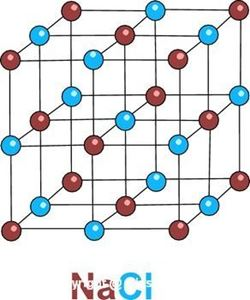This is a basic introductory chemistry course designed for 10th grade students who already have a little bit of physical science background and who have completed algebra 1. We cover a wide range of topics, starting with a review unit called scientific foundations. We then move on to cover units on states of matter, atomic structure, periodic table, bnoding and naming, reactions, and stoichiometry. By the end of the course, you will be prepared to enroll in either a second year high school chemistry course or have the basics needed for college admission and with the background needed to do well in chemistry in that setting. There are over 12 hours of video content covering 22 lessons in this course, each with a lesson video, worksheet, worksheet review video, and quiz. Are you ready to learn some chemistry?

#### 1 Lesson 1: Metric System and Dimensional Analysis 20:51In this lesson we take a quick review of the metric system, focusing on the units and measurements needed for the successful study of chemistry. We look at how to convert within the metric system by simply moving the decimal point. Then we progress from there to another method of making the same conversion and which will also allow us to make conversions that require more than just sliding the decimal point. The objectives are to recognize the basi units of measure and their abbreviations, to use and recognize the most commonly used prefixes in the metric system, and to use the technique of dimensional analysis to convert from one type of unit to another.

#### 2 Lesson 1 Review 16:39This lesson reviews the worksheet that correlates with the lesson on Metric System and Dimensional Analysis.

#### 3 Lesson 2: Significant Figures 19:14In this lesson we discuss uncertainty in measurement as well as accuracy and precision in measurement. We build on these ideas to come up with a basis for using only those digits in numbers which are significant. The objectives are to understand these terms of uncertainty, accuracy, and precision; to determine the number of significant figures in a value; and to use basic rules for determining significant figures in results once a mathematical operation (add/subtract/multiply/divide) has been performed.

#### 4 Lesson 2 Review 08:50This is the review lesson that goes over the worksheet from the Significant Figures lesson.

#### 5 Lesson 3: Scientific Notation 18:21In this lesson we look at how numbers large or small are converted into scientific notation and then learn the rules for performing mathematical operations on numbers written in scientific notation. The objectives are to be able to write either large or small numbers in correct scientific notation format, to correctly perform addition or subtraction on those values, to correctly perform multiplication or division on those values, and to recognize when results of calculations need to be adjusted to obtain proper format and/or to ensure the correct number of significant figures.

#### 6 Lesson 3 Review 15:21This lesson reviews the worksheet that accompanies the lesson on Scientific Notation.

#### 7 Lesson 4: Physical and Chemical Properties and Changes 21:09This lesson looks at physical properties, chemical properties, physical changes, and chemical changes. It meets four main objectives: distinguishing between a mixture and a pure substance, distinguishing between physical properties and chemical properties, distinguishing between extensive and intensive physical properties, and classifying changes in matter as physical and chemical.

#### 8 Lesson 4 Review 06:32In this video the worksheet from the lesson on Physical and Chemical Properties and Changes is reviewed.

#### 9 Lesson 5: Changes in State and the Phase Diagram 26:29The topic for this lesson is Changes in State and the Phase Diagram. In it, we cover the objectives of describing the three main states of matter in terms of kinetic molecular theory; identifying the 6 changes in state of solids, liquids, and gases; understanding what causes boiling and how boiling point is affected by atmospheric pressure; and reading and interpreting phase diagrams.

#### 10 Lesson 5 Review 10:07This is a review of the worksheet that accompanies the lesson on Changes in State and the Phase Diagram.

#### 11 Lesson 6: History of Atomic Theory 08:44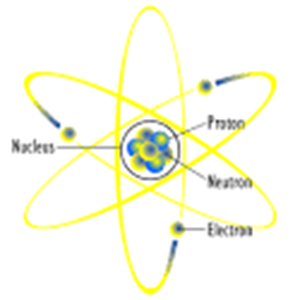This lesson reviews the advances in our understanding of the atom and the evolution of atomic models and atomic theory. Its main objectives are to examine how understanding of the atom has progressed over time with advances in technology and to understand the basic components (subatomic particles) and their arrangement in the atom.

#### 12 Lesson 6 Review 05:57This video reviews the worksheet corresponding to the lesson History of Atomic Theory.

#### 13 Lesson 7: The Atom 22:28In this lesson, the stucture of an atom is reviewed and we start to use the periodic table to tell us thigs about a particular atom of a particular element. The lesson objectives are to identify the subatomic particles and their locations in an atom; to use an element key; to identify the number of protons, neutrons, and electrons a given element has; to differentiate isotopes of the same element (nuclides); and to learn hyphen notation and nuclear symbol notation to write isotopes.

#### 14 Lesson 7 Review 10:47This video reviews the worksheet that accompanies the lesson The Atom.

#### 15 Lesson 8: Electron Configuration 25:27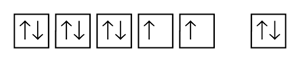This lesson introduces the student to the concept of electron configuration. The objectives are to understand the concept of quantum numbers to be able to follow Aufbau Principle, Pauli Exclusion Principle, and Hund's Rule in the filling of orbitals; to differentiate the characteristics of s, p, d, and f orbitals that are essential to electron notation; to be able to use the periodic table as a guide in filling order for electron notation; and to be familiar with and comfortable with the three methods of writing electron configuration (orbital notation, electron configuration notation, and noble gas notation).

#### 16 Lesson 8 Review 13:30This video reviews information on the worksheet for the lesson Electron Configuration.

#### 17 Lesson 9: Periodic Table 18:49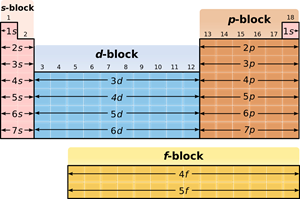The objectives for this lesson are to understand the arrangement of the periodic table in terms of specific groups of elements (metals, nonmetals, metalloids, alkali metals, alkaline earth metals, etc.), to differentiate the properties of each of these separate groups, and to make the connection between placement on the periodic table, electron configuration, and reactivity.

#### 18 Lesson 9 Review 18:22This is the worksheet review video for the Periodic Table.

#### 19 Lesson 10: Periodicity 10:53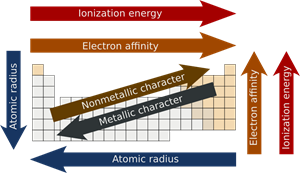This lesson is focused on detailed review of periodicity. The objectives are to understand what causes periodicity; to understand the trends seen in atomic radii, ionization energy, electron affinity, and electronegativity; and to understand the role of valence electrons in periodicity and chemical bonding.

#### 20 Lesson 10 Review 06:47This is the review of the worksheet for the Periodicity lesson.

#### 21 Lesson 11: Types of Chemical Bonds 26:34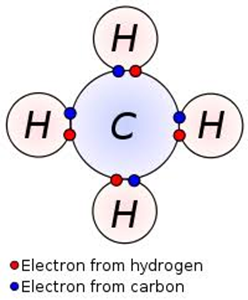The focus of this lesson is on the main types of chemical bonds and their subtypes as well as what causes each of the different types to form and what properties result from a particular type of bond. The objectives are to understand what a chemical bond is, to differentiate between ionic and covalent bonds, to differentiate between polar and nonpolar covalent bonds, to understand the properties that result with each type of bond, and to evaluate each bond in a compound and determien what type of bond it is based on electronegativity of the atoms involved.

#### 22 Lesson 11 Review 10:24This lesson is a review of the worksheet for the Types of Chemical Bonds lesson.

#### 23 Lesson 12: Lewis Structures 26:38This lesson shows the techniques of dot diagrams and Lewis structures, building on previous lessons of electron configuration, periodicity, and chemical bonds. The objectives are to understand and use the correct method for creating dot diagrams of individual atoms, understand and use the correct method for creating Lewis structures of compounds, and use the dot diagrams to predict stability of atoms and numbers of bonds that can be formed in chemical compounds.

#### 24 Lesson 12 Review 13:49This video reviews the worksheet accompanying the lesson on Lewis Structures.

#### 25 Lesson 13: Intermolecular Forces 16:13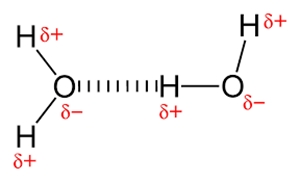In this lesson we look at the various types of attractive forces that are found not within a single molecule but between two or more molecules. The objectives for this lesson are to understand what intermolecular forces are, how intermolecular forces affect boiling and melting points of molecules, to determine the polarity of a molecule, and to differentiate the main types of intermolecular forces: dipole-dipole, induced dipole, hydrogen bonds, and London dispersion forces.

#### 26 Lesson 13 Review 08:33This video reviews the answers on the worksheet for the lesson Intermolecular Forces.

#### 27 Lesson 14: Writing and Naming Ionic Compounds, part 1 25:33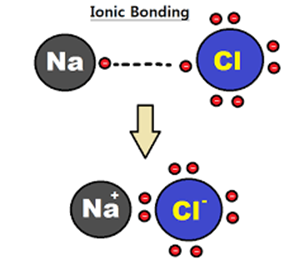In this lesson we begin learning how to write and name ionic compounds, beginning with the most basic rules and simple binary compounds. The objectives are to understand and interpret chemical formulas, to understand the basic rules for how to name an ionic compound, to learn how to calculate the charges of ions in a compound and to work the other way and determine the ratio of elements when writing a chemical formula, to practice naming binary ionic compounds, and to use the stock system for both how to write a formula when given the name and how to name a compound when given the formula.

#### 28 Lesson 14 Review 15:32This lesson reviews the worksheet that accompanies part 1 of the lesson on Writing and Naming Ionic Compounds.

#### 29 Lesson 15: Writing and Naming Ionic Compounds, part 2 18:20In this lesson we begin learning how to write and name ionic compounds, beginning with the most basic rules and simple binary compounds. The objectives are to understand and interpret chemical formulas, to understand the basic rules for how to name an ionic compound, to learn how to calculate the charges of ions in a compound and to work the other way and determine the ratio of elements when writing a chemical formula, to practice naming polyatomic ionic compounds, and to use the stock system for both how to write a formula when given the name and how to name a compound when given the formula. The emphasis on all of these objectives will be with newly defined polyatomic ions.

#### 30 Lesson 15 Review 11:51This lesson is a review of part 2 of the topic Writing and Naming Ionic Compounds and focuses on polyatomic ions.

#### 31 Lesson 16: Writing and Naming Molecular Compounds 14:26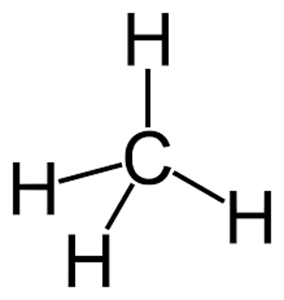This lesson builds on the previous topic of writing and naming ionic compounds, now focusing on writing and naming molecular compounds, or those held together by covalent bonds. The objectives are similar: understand the format used for naming binary molecular compounds, know when to use and when not to use prefixes, understand that the prefixes in no way tell the charge of the elements involved, write formulas for compounds when given their names, and write names of compounds when given their formulas.

#### 32 Lesson 16 Review 09:10This is the worksheet review portion of the lesson on Writing and Naming Molecular Compounds.

#### 33 Lesson 17: Chemical Reactions 29:25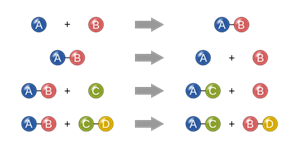In this lesson we go into detail on what a chemical reaction is and how to write and balance them. The objectives are to identify indicators of possible chemical change, to apply Law of Conservation of Mass in a chemical equation, to write a chemical equation given that equation in word form including knowing when diatomic elements apply, to understand the use of symbols in a chemical equation, and to balance chemical equations.

#### 34 Lesson 17 Review 15:31This lesson is the worksheet review that coordinates with the lesson of the same name.

#### 35 Lesson 18: Types of Reactions 24:20In this lesson, we look at the different types of chemical reactions and how to identify each one. The objectives are to identify the 5 main types of chemical reactions, to understand the generalized formulas showing each one, and to be able to predict the products formed when reactants and type of rection are given.

#### 36 Lesson 18 Review 07:17This lesson reviews the worksheet for the lesson Types of Chemical Reactions.

#### 37 Lesson 19: Net Ionic Equations and Solubility 24:28This lesson builds on previous lessons where we learned how to write chemical formulas, how to balance equations, and how to identify types of chemical reactions. We take all of those aspects combined and add in how to determine whether a compound formed is soluble or not and then how to write what's called the net ionic equation. So our objectives are to understand the new terminology involved, to identify whether or not a compound is soluble based on a table of solubilities (provided), to write an ionic equation, to identify spectator ions, and finally, to write a correct and balanced net ionic equation.

#### 38 Lesson 19 Review 16:25This lesson reviews the worksheet accompanying the Net Ionic Equations lesson.

#### 39 Lesson 20: The Mole 27:14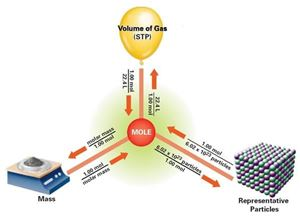This lesson introduces the concept of the mole and teaches students how to make the necessary conversions to and from the mole. We build on previous lesson of writing chemical formulas, writing a balanced formula equation, and using dimensional analysis with significant figures and scientific notation. The objectives are to understand what the mole is and its abbreviation, to understand and use Avogadro's number, to understand the relationships between mole, number of molecules, mass, and volume of the gaseous form of a substance, and to apply these relationships to calculate quntities of each when given one value.

#### 40 Lesson 20 Review 15:14This lesson contains the review video for the worksheet accompanying the lesson The Mole.

#### 41 Lesson 21: Stoichiometry 23:27This lesson builds on the previous lesson of The Mole. In this lesson, those same concepts are applied with the added layer that we can convert between different substances at the mole level using a balanced chemical equation. The objectives are to calculate theoretical yields or starting materials given a quantity of one component of the reaction and calculate percent yield based on calculation of theoretical yield and a given quantity of actual yield.

#### 42 Lesson 21 Review 14:26This lesson reviews the worksheet accompanying the lesson on Stoichiometry.

#### 43 Lesson 22: Limiting Reagent 28:44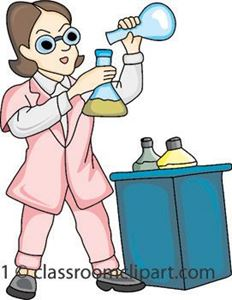In this lesson we use the skills learned in stoichiometry to determine the limiting reagent for a reaction, as now we will have given quantities for both reactants rather than starting amount of one reactant and unlimited second reactant. Once we know limiting reagent, we can then determine how much of the excess reactant will be left over and calculate the theoretical yield of any products. The objectives are to determine limiting reagent, to calculate the excess reactant, and to calculate theoretical yield.

#### 44 Lesson 22 Review 23:44This is the review lesson for Limiting Reagent in which the answers to the worksheet are reviewed.

Course Description

This is a basic introductory chemistry course suitable for students with a little bit of physical science background. We cover a range of relevant topics from basic structure of the atom and the history of atomic theory, through writing and balancing chemical equtions, and finally all the way up to my favorite subject, stoichiometry, where we tie all of that background knowledge together and apply it to the idea of limiting reagents.

Course Goals

Upon completion of this course, students will have the background they need to be successful in a 2nd year of high school chemistry at the 11th or 12th grade level. This course will also provide the background necessary for students who wish to progress to a college or university.

Target Audience

This course is designed for students with some physical science background at the middle school level. It is also essential that students have a solid understanding of algebra.

Course Includes

7 Units and 44 video lessons

22 Engaging video-lessons with practice worksheets

22 Worksheet review videos (I explain step by step the correct answer)

22 Quizzes

22 Quiz Answer Keys (for parents)

Over 12 hours of video!

Course Content

This course is broken up into 22 lesson and contains over 12 hours of video content. Each lesson include the lesson video, a corresponding practice worksheet, a second video to review that video, and a quiz to ensure you have a solid grasp on the lesson. The lessons included are the following:

1: Metric System and Dimensional Analysis

2: Significant Figures

3: Scientific Notation

4: Physical and Chemical Properties and Changes

5: Changes in State and the Phase Diagram

6: History of Atomic Theory

7: The Atom

8: Electron Configuration

9: Periodic Table

10: Periodicity

11: Types of Chemical Bonds

12: Lewis Structures

13: Intermolecular Forces

14: Writing and Naming Ionic Componds

15: Writing and Naming Ionic Componds, part 2

16: Writing and Naming Covalent Molecules

17: Chemical Reactions

18: Types of Chemical Reactions

19: Net Ionic Equations

20: The Mole (hint: it's not a furry rodent!)

21: Stoichiometry

22: Limiting Reagent

• Teacher: Kristi
• Areas of expertise: Chemistry, Biology, Marine Biology, Forensic Toxicology, Environmental Science
• Education: Bachelor of Science in Marine Biology, Master of Science in “Wildlife and Fisheries Sciences” with an emphasis in environmental science education, Master of Science in Forensic Toxicology
• Interests: spending time outdoors, camping, hiking, reading, knitting
• Skills: research lab experience; AP Biology trained; experienced physical science, chemistry, biology, and AP biology teacher
• Associations: Certified teacher in the state of Florida, subjects include Biology 6-12, Chemistry 6-12, and Math 5-9. Gifted Endorsed.
• Issues I care about: Scientific literacy, quality education, environmental health and safety

Hello! I’m Kristi. I am a middle and high school science teacher with lab experience and a history of working as an EMT and camp health manager. I am a certified teacher in the State of Florida for the following subjects: Biology, Chemistry, and middle grades math. In my seven years of teaching, I have taught physical science, life science, algebra I, biology, ecology, chemistry honors, and AP Biology. When I was in high school, I absolutely loved learning about the courses I am now teaching, and I enjoy sharing that passion with my students. My students often tell me I make class enjoyable (even when they don’t particularly like science) and that they find it easy to learn from the way I teach. I hope you feel the same way!

This document contains all the correct answers for all the tests which appear at the end of each lesson.

#### Lesson 1 answer key, Metric System and Dimensional Analysis

In this file are the answers for the Metric System and Dimensional Analysis worksheet. For complete explanation of the answers, please watch the corresponding lesson review video.

#### Lesson 2 answer key, Significant Figures

In this file are the answers for the Significant Figures worksheet. For complete explanation of the answers, please watch the corresponding lesson review video.

#### Lesson 3 answer key, Scientific Notation

In this file are the answers for the Scientific Notation worksheet. For complete explanation of the answers, please watch the corresponding lesson review video.

#### Lesson 4 answer key, Physical and Chemical Properties and Changes

In this file are the answers for the Physical and Chemical Properties and Changes worksheet. For complete explanation of the answers, please watch the corresponding lesson review video.

#### Lesson 5 answer key, Changes in State and the Phase Diagram

In this file are the answers for the Changes in State and the Phase Diagram worksheet. For complete explanation of the answers, please watch the corresponding lesson review video.

#### Lesson 6 answer key, History of Atomic Theory

In this file are the answers for the History of Atomic Theory worksheet. For complete explanation of the answers, please watch the corresponding lesson review video.

#### Lesson 7 answer key, The Atom

In this file are the answers for The Atom worksheet. For complete explanation of the answers, please watch the corresponding lesson review video.

#### Lesson 8 answer key, Electron Configuration

In this file are the answers for the Electron Configuration worksheet. For complete explanation of the answers, please watch the corresponding lesson review video.

#### Lesson 9 answer key, Periodic Table

In this file are the answers for the Periodic Table worksheet. For complete explanation of the answers, please watch the corresponding lesson review video.

#### Lesson 10 answer key, Periodicity

In this file are the answers for the Periodicity worksheet. For complete explanation of the answers, please watch the corresponding lesson review video.

#### Lesson 11 answer key, Types of Chemical Bonds

In this file are the answers for the Types of Chemical Bonds worksheet. For complete explanation of the answers, please watch the corresponding lesson review video.

#### Lesson 12 answer key, Lewis Structures

In this file are the answers for the Lewis Structures worksheet. For complete explanation of the answers, please watch the corresponding lesson review video.

#### Lesson 13 answer key, Intermolecular Forces

In this file are the answers for the Intermolecular Forces worksheet. For complete explanation of the answers, please watch the corresponding lesson review video.

#### Lesson 14 answer key, Writing and Naming Ionic Compounds, part 1

In this file are the answers for the Writing and Naming Ionic Compounds, part 1 worksheet. For complete explanation of the answers, please watch the corresponding lesson review video.

#### Lesson 15 answer key, Writing and Naming Ionic Compounds, part 2

In this file are the answers for the Writing and Naming Ionic Compounds, part 2 worksheet. For complete explanation of the answers, please watch the corresponding lesson review video.

#### Lesson 16 answer key, Writing and Naming Molecular Compounds

In this file are the answers for the Writing and Naming Molecular Compounds worksheet. For complete explanation of the answers, please watch the corresponding lesson review video.

#### Lesson 17 answer key, Chemical Reactions

In this file are the answers for the Chemical Reactions worksheet. For complete explanation of the answers, please watch the corresponding lesson review video.

#### Lesson 18 answer key, Types of Chemical Reactions

In this file are the answers for the Types of Chemical Reactions worksheet. For complete explanation of the answers, please watch the corresponding lesson review video.

#### Lesson 19 answer key, Net Ionic Equations

In this file are the answers for the Net Ionic Equations worksheet. For complete explanation of the answers, please watch the corresponding lesson review video.

#### Lesson 20 answer key, The Mole

In this file are the answers for The Mole worksheet. For complete explanation of the answers, please watch the corresponding lesson review video.

#### Lesson 21 answer key, Stoichiometry

In this file are the answers for the Stoichiometry worksheet. For complete explanation of the answers, please watch the corresponding lesson review video.

#### Lesson 22 answer key, Limiting Reagent

In this file are the answers for the Limiting Reagent worksheet. For complete explanation of the answers, please watch the corresponding lesson review video.

Customers who bought this item also boughtThis course breaks down how to write an engaging, clear, logical paragraph. It covers a variety of paragraph types and helps you learn how to structure a paragraph so that it best conveys your message to your readers. +
\$150.00 \$74.00